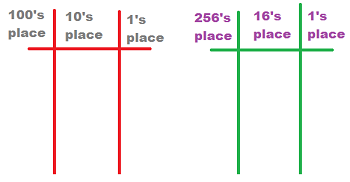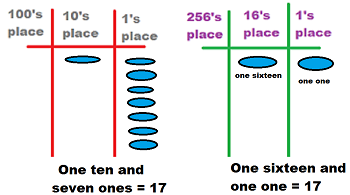# What is a Hexadecimal? - Definition & Conversion

Instructor: Mark Boster
We use a base-ten system to count and solve math problems. However, if you would like to program computers, you need to learn about hexadecimals, a number system based on sixteen.

Computer scientists create the computer systems that you use to surf the net, play games online, and even read online lessons like these. And they program computers using a number system called hexadecimal.

A hexadecimal is a number system based on the number sixteen. To remember this, remember that hexa means six (like how a hexagon has six sides), and decimal refers to a system based on the number ten. And what do you get when you add six and ten? Sixteen!

So, instead of having only ten single-digit numbers (0 through 9), a hexadecimal system has sixteen single-digit numbers. These are the sixteen single-digit numbers of the hexadecimal system:

• 0, 1 , 2, 3, 4, 5, 6, 7, 8, 9, A, B, C, D, E, F

Because there are only so many single-digit numbers in math, the system uses some letters as numbers. In the hexadecimal system:

• A is 10
• B is 11
• C is 12
• D is 13
• E is 14
• F is 15

The number system we use every day is base-ten, and its place values are the ones column, tens column (which represents the numeral x 10), hundreds column (which represents the numeral x 10 x 10), and so on. After the ones place, each place value increases by the power of 10.In the hexadecimal (base-sixteen) system, there are also place values, but they are different. It starts with the ones, and each place value increases by the power of 16. So, there's a ones column, a sixteens column (which represents the numeral x 16), a two-hundred-and-fifty-sixes column (which represents the numeral x 16 x 16), and so on.

#### Comparing Base-Ten and Base-Sixteen Place Values

Let's use the number 17 to compare the base-ten system and the hexadecimal (base-sixteen) system.Take a look at the base-ten chart. In the ones place, you can only go up to 9. After that--to represent a ten--you have to move over to the tens place. So, to show 17, you make one mark in the tens place column and seven marks in the ones place column.

To unlock this lesson you must be a Study.com Member.

### Register to view this lesson

Are you a student or a teacher?

#### See for yourself why 30 million people use Study.com

##### Become a Study.com member and start learning now.
Back
What teachers are saying about Study.com

### Earning College Credit

Did you know… We have over 200 college courses that prepare you to earn credit by exam that is accepted by over 1,500 colleges and universities. You can test out of the first two years of college and save thousands off your degree. Anyone can earn credit-by-exam regardless of age or education level.